Next: Plasma Parameter Up: Introduction Previous: Plasma Frequency

# Debye Shielding

Plasmas generally do not contain strong electric fields in their rest frames. The shielding of an external electric field from the interior of a plasma can be viewed as a result of high plasma conductivity: i.e., plasma current generally flows freely enough to short out interior electric fields. However, it is more useful to consider the shielding as a dielectric phenomena: i.e., it is the polarization of the plasma medium, and the associated redistribution of space charge, which prevents penetration by an external electric field. Not surprisingly, the length-scale associated with such shielding is the Debye length.

Let us consider the simplest possible example. Suppose that a quasi-neutral plasma is sufficiently close to thermal equilibrium that its particle densities are distributed according to the Maxwell-Boltzmann law,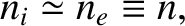(11)

whereis the electrostatic potential, andand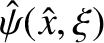are constant. From, it is clear that quasi-neutrality requires the equilibrium potential to be a constant. Suppose that this equilibrium potential is perturbed, by an amount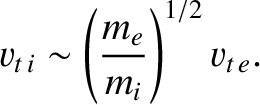, by a small, localized charge density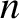. The total perturbed charge density is written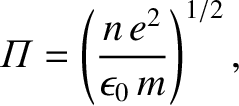(12)

Thus, Poisson's equation yields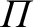(13)

which reduces to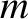(14)

If the perturbing charge density actually consists of a point charge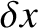, located at the origin, so that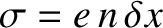, then the solution to the above equation is written(15)

Clearly, the Coulomb potential of the perturbing point chargeis shielded on distance scales longer than the Debye length by a shielding cloud of approximate radiusconsisting of charge of the opposite sign.

Note that the above argument, by treating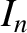as a continuous function, implicitly assumes that there are many particles in the shielding cloud. Actually, Debye shielding remains statistically significant, and physical, in the opposite limit in which the cloud is barely populated. In the latter case, it is the probability of observing charged particles within a Debye length of the perturbing charge which is modified.Next: Plasma Parameter Up: Introduction Previous: Plasma Frequency
Richard Fitzpatrick 2011-03-31